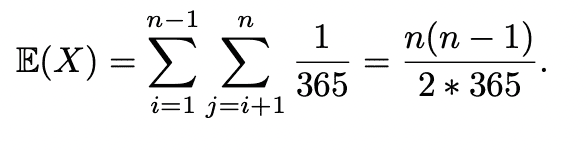# Trouble with a double summation

docnet
Homework Statement:
verify the last equality
Relevant Equations:
verify the last equalitythe inner sum is just adding 1/365 n-i number of times. so ##\frac{(n-i)}{365}##

the outer sum adds over the index i, so I thought the expression is equal to ##\frac{(n-1)n-(n-1)!}{365}## but it's obviously not equal to that. where did I go wrong?

Homework Helper
Gold Member
2022 Award
Homework Statement:: verify the last equality
Relevant Equations:: verify the last equality

View attachment 291798

the inner sum is just adding 1/365 n-i number of times. so ##\frac{(n-i)}{365}##

the outer sum adds over the index i, so I thought the expression is equal to ##\frac{(n-1)n-(n-1)!}{365}## but it's obviously not equal to that. where did I go wrong?
If ##i = 1## there are ##n-1## terms, if ##i =2## there are ##n -2## terms etc. Hence there are ##1 +2 \dots + n -1## terms in total.

Do an example with ##n = 4## say, and write out the sum.

•docnet
docnet
Homework Statement:: verify the last equality
Relevant Equations:: verify the last equality

View attachment 291798

the inner sum is just adding 1/365 n-i number of times. so ##\frac{(n-i)}{365}##

the outer sum adds over the index i, so I thought the expression is equal to ##\frac{(n-1)n-(n-1)!}{365}## but it's obviously not equal to that. where did I go wrong?
If ##i = 1## there are ##n-1## terms, if ##i =2## there are ##n -2## terms etc. Hence there are ##1 +2 \dots + n -1## terms in total.

Do an example with ##n = 4## say, and write out the sum.
If ##n=4##, then the sum is

##1/365\cdot \sum^3_{i=1}\sum^4_{j=i+1}1=1/365\cdot (3+2+1)=\frac{6}{365}##.

check:
##\frac{n(n-1)}{2*365}=\frac{4(3)}{2*365}=\frac{6}{365}##

so I realized that I forgot the meaning of the factorial.. oope. I somehow thought it was n! = n+(n-1)+...+2+1 . oh lord.

following the same thought process suggested by @PeroK,

##1/365\cdot sum^{n-1}_{i=1}\sum^n_{j=i+1}1=\frac{(n-1)+(n-2)+...+2+1}{365}##

which is equivalent to ##\frac{n(n-1)}{2*365}## (although I don't know how to prove it, I can see that it is true geometrically.)

Gold Member
$$\sum_{i=1}^{n-1}\sum_{j=i+1}^{n}1=\sum_{i=1}^{n-1}(n-i)=n\sum_{i=1}^{n-1}1-\sum_{i=1}^{n-1}i=n(n-1)-\sum_{i=1}^{n-1}i$$
Then
$$S:=\sum_{i=1}^{n-1}i=\sum_{i=1}^{n-1}(n-i)$$
which means just changing order of addition
$$1+2+...+(n-1)=(n-1)+(n-2)+...+2+1$$
$$2S=\sum_{i=1}^{n-1}n=n\sum_{i=1}^{n-1}1=n(n-1)$$
This is equivalent to geometrical explanation.

••SammyS and docnet
docnet
$$S:=\sum_{i=1}^{n-1}i=\sum_{i=1}^{n-1}(n-i)$$
This confused me at first, but it makes sense after thinking about it. Thank you so much.

•anuttarasammyak